### Learning Objectives

• Describe the linear approximation to a function at a point.
• Write the linearization of a given function.
• Draw a graph that illustrates the use of differentials to approximate the change in a quantity.
• Calculate the relative error and percentage error in using a differential approximation.

We have just seen how derivatives allow us to compare related quantities that are changing over time. In this section, we examine another application of derivatives: the ability to approximate functions locally by linear functions. Linear functions are the easiest functions with which to work, so they provide a useful tool for approximating function values. In addition, the ideas presented in this section are generalized in the second volume of this text, when we studied how to approximate functions by higher-degree polynomials in the  Introduction to Power Series and Functions .

# Linear Approximation of a Function at a Point

Consider a function $f$ that is differentiable at a point $x=a$. Recall that the tangent line to the graph of $f$ at $a$ is given by the equation

$y=f(a)+f^{\prime}(a)(x-a)$.

For example, consider the function $f(x)=\frac{1}{x}$ at $a=2$. Since $f$ is differentiable at $x=2$ and $f^{\prime}(x)=-\frac{1}{x^2}$, we see that $f^{\prime}(2)=-\frac{1}{4}$. Therefore, the tangent line to the graph of $f$ at $a=2$ is given by the equation

$y=\frac{1}{2}-\frac{1}{4}(x-2)$.

(Figure) (a) shows a graph of $f(x)=\frac{1}{x}$ along with the tangent line to $f$ at $x=2$. Note that for $x$ near 2, the graph of the tangent line is close to the graph of $f$. As a result, we can use the equation of the tangent line to approximate $f(x)$ for $x$ near 2. For example, if $x=2.1$, the $y$ value of the corresponding point on the tangent line is

$y=\frac{1}{2}-\frac{1}{4}(2.1-2)=0.475$.

The actual value of $f(2.1)$ is given by

$f(2.1)=\frac{1}{2.1}\approx 0.47619$.

Therefore, the tangent line gives us a fairly good approximation of $f(2.1)$ ( (Figure) (b)). However, note that for values of $x$ far from 2, the equation of the tangent line does not give us a good approximation. For example, if $x=10$, the $y$-value of the corresponding point on the tangent line is

$y=\frac{1}{2}-\frac{1}{4}(10-2)=\frac{1}{2}-2=-1.5$,

whereas the value of the function at $x=10$ is $f(10)=0.1$.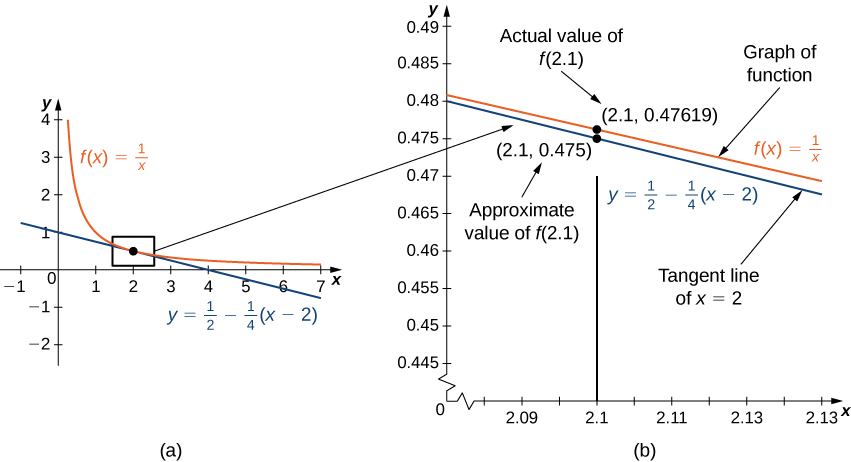Figure 1. (a) The tangent line to $f(x)=1/x$ at $x=2$ provides a good approximation to $f$ for $x$ near 2. (b) At $x=2.1$, the value of $y$ on the tangent line to $f(x)=1/x$ is 0.475. The actual value of $f(2.1)$ is $1/2.1$, which is approximately 0.47619.

In general, for a differentiable function $f$, the equation of the tangent line to $f$ at $x=a$ can be used to approximate $f(x)$ for $x$ near $a$. Therefore, we can write

$f(x)\approx f(a)+f^{\prime}(a)(x-a)$ for $near [latex]a$.

We call the linear function

$L(x)=f(a)+f^{\prime}(a)(x-a)$

the linear approximation , or tangent line approximation , of $f$ at $x=a$. This function $L$ is also known as the linearization of $f$ at $x=a$.

To show how useful the linear approximation can be, we look at how to find the linear approximation for $f(x)=\sqrt{x}$ at $x=9$.

### Linear Approximation of $\sqrt{x}$

Find the linear approximation of $f(x)=\sqrt{x}$ at $x=9$ and use the approximation to estimate $\sqrt{9.1}$.

#### Solution

Since we are looking for the linear approximation at $x=9$, using (Figure) we know the linear approximation is given by

$L(x)=f(9)+f^{\prime}(9)(x-9)$.

We need to find $f(9)$ and $f^{\prime}(9)$.

$\begin{array}{lll} f(x)=\sqrt{x}& \Rightarrow & f(9)=\sqrt{9}=3 \\ f^{\prime}(x)=\frac{1}{2\sqrt{x}}& \Rightarrow & f^{\prime}(9)=\frac{1}{2\sqrt{9}}=\frac{1}{6} \end{array}$

Therefore, the linear approximation is given by (Figure) .

$L(x)=3+\frac{1}{6}(x-9)$

Using the linear approximation, we can estimate $\sqrt{9.1}$ by writing

$\sqrt{9.1}=f(9.1)\approx L(9.1)=3+\frac{1}{6}(9.1-9)\approx 3.0167$.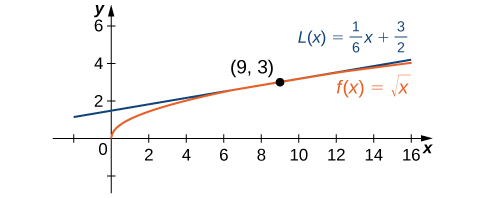Figure 2. The local linear approximation to $f(x)=\sqrt{x}$ at $x=9$ provides an approximation to $f$ for $x$ near 9.

#### Analysis

Using a calculator, the value of $\sqrt{9.1}$ to four decimal places is 3.0166. The value given by the linear approximation, 3.0167, is very close to the value obtained with a calculator, so it appears that using this linear approximation is a good way to estimate $\sqrt{x}$, at least for $x$ near 9. At the same time, it may seem odd to use a linear approximation when we can just push a few buttons on a calculator to evaluate $\sqrt{9.1}$. However, how does the calculator evaluate $\sqrt{9.1}$? The calculator uses an approximation! In fact, calculators and computers use approximations all the time to evaluate mathematical expressions; they just use higher-degree approximations.

Find the local linear approximation to $f(x)=\sqrt{x}$ at $x=8.$ Use it to approximate $\sqrt{8.1}$ to five decimal places.

#### Hint

$L(x)=f(a)+{f}^{\prime } (a)(x-a)$

#### Solution

$L(x)=2+\frac{1}{12}(x-8);$ 2.00833

### Linear Approximation of $\sin x$

Find the linear approximation of $f(x)= \sin x$ at $x=\frac{\pi }{3}$ and use it to approximate $\sin (62\text{°}).$

#### Solution

First we note that since $\frac{\pi }{3}$ rad is equivalent to $60\text{°},$ using the linear approximation at $x=\pi \text{/}3$ seems reasonable. The linear approximation is given by

$L(x)=f(\frac{\pi }{3})+{f}^{\prime } (\frac{\pi }{3})(x-\frac{\pi }{3}).$

We see that

$\begin{array}{ccc}\hfill f(x)= \sin x& ⇒\hfill & f(\frac{\pi }{3})= \sin (\frac{\pi }{3})=\frac{\sqrt{3}}{2}\hfill \\ \hfill {f}^{\prime } (x)= \cos x& ⇒\hfill & {f}^{\prime } (\frac{\pi }{3})= \cos (\frac{\pi }{3})=\frac{1}{2}\hfill \end{array}$

Therefore, the linear approximation of $f$ at $x=\pi \text{/}3$ is given by (Figure) .

$L(x)=\frac{\sqrt{3}}{2}+\frac{1}{2}(x-\frac{\pi }{3})$

To estimate $\sin (62\text{°})$ using $L,$ we must first convert 62° to radians. We have $62\text{°}=\frac{62\pi }{180}$ radians, so the estimate for $\sin (62\text{°})$ is given by

$\sin (62\text{°})=f(\frac{62\pi }{180})\approx L(\frac{62\pi }{180})=\frac{\sqrt{3}}{2}+\frac{1}{2}(\frac{62\pi }{180}-\frac{\pi }{3})=\frac{\sqrt{3}}{2}+\frac{1}{2}(\frac{2\pi }{180})=\frac{\sqrt{3}}{2}+\frac{\pi }{180}\approx 0.88348.$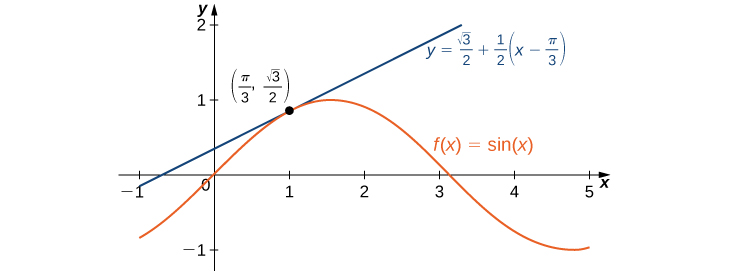Figure 3. The linear approximation to $f(x)= \sin x$ at $x=\pi \text{/}3$ provides an approximation to $\sin x$ for $x$ near $\pi \text{/}3.$

Find the linear approximation for $f(x)= \cos x$ at $x=\frac{\pi }{2}.$

#### Hint

$L(x)=f(a)+{f}^{\prime } (a)(x-a)$

#### Solution

$L(x)=-x+\frac{\pi }{2}$

Linear approximations may be used in estimating roots and powers. In the next example, we find the linear approximation for $f(x)={(1+x)}^{n}$ at $x=0,$ which can be used to estimate roots and powers for real numbers near 1. The same idea can be extended to a function of the form $f(x)={(m+x)}^{n}$ to estimate roots and powers near a different number $m.$

### Approximating Roots and Powers

Find the linear approximation of $f(x)={(1+x)}^{n}$ at $x=0.$ Use this approximation to estimate ${(1.01)}^{3}.$

#### Solution

The linear approximation at $x=0$ is given by

$L(x)=f(0)+{f}^{\prime } (0)(x-0).$

Because

$\begin{array}{ccc}\hfill f(x)={(1+x)}^{n}& ⇒\hfill & f(0)=1\hfill \\ \hfill {f}^{\prime } (x)=n{(1+x)}^{n-1}& ⇒\hfill & {f}^{\prime } (0)=n,\hfill \end{array}$

the linear approximation is given by (Figure) (a).

$L(x)=1+n(x-0)=1+nx$

We can approximate ${(1.01)}^{3}$ by evaluating $L(0.01)$ when $n=3.$ We conclude that

${(1.01)}^{3}=f(1.01)\approx L(1.01)=1+3(0.01)=1.03.$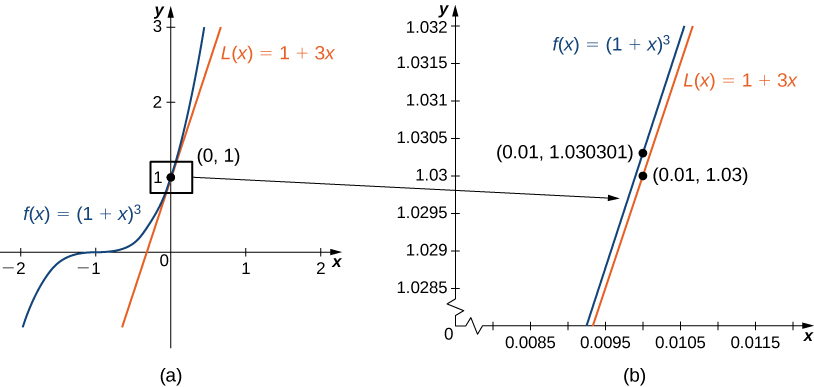Figure 4. (a) The linear approximation of $f(x)$ at $x=0$ is $L(x).$ (b) The actual value of ${1.01}^{3}$ is 1.030301. The linear approximation of $f(x)$ at $x=0$ estimates ${1.01}^{3}$ to be 1.03.

Find the linear approximation of $f(x)={(1+x)}^{4}$ at $x=0$ without using the result from the preceding example.

#### Hint

${f}^{\prime } (x)=4{(1+x)}^{3}$

#### Solution

$L(x)=1+4x$

# Differentials

We have seen that linear approximations can be used to estimate function values. They can also be used to estimate the amount a function value changes as a result of a small change in the input. To discuss this more formally, we define a related concept: differentials. Differentials provide us with a way of estimating the amount a function changes as a result of a small change in input values.

When we first looked at derivatives, we used the Leibniz notation $dy\text{/}dx$ to represent the derivative of $y$ with respect to $x.$ Although we used the expressions dy and dx in this notation, they did not have meaning on their own. Here we see a meaning to the expressions dy and dx . Suppose $y=f(x)$ is a differentiable function. Let dx be an independent variable that can be assigned any nonzero real number, and define the dependent variable $dy$ by

$dy={f}^{\prime } (x)dx.$

It is important to notice that $dy$ is a function of both $x$ and $dx.$ The expressions dy and dx are called differentials . We can divide both sides of (Figure) by $dx,$ which yields

$\frac{dy}{dx}={f}^{\prime } (x).$

This is the familiar expression we have used to denote a derivative. (Figure) is known as the differential form of (Figure) .

### Computing differentials

For each of the following functions, find dy and evaluate when $x=3$ and $dx=0.1.$

1. $y={x}^{2}+2x$
2. $y= \cos x$

#### Solution

The key step is calculating the derivative. When we have that, we can obtain dy directly.

1. Since $f(x)={x}^{2}+2x,$ we know ${f}^{\prime } (x)=2x+2,$ and therefore
$dy=(2x+2)dx.$

When $x=3$ and $dx=0.1,$

$dy=(2\cdot3+2)(0.1)=0.8.$
2. Since $f(x)= \cos x,$ ${f}^{\prime } (x)=- \sin (x).$ This gives us
$dy=- \sin xdx.$

When $x=3$ and $dx=0.1,$

$dy=- \sin (3)(0.1)=-0.1 \sin (3).$

For $y={e}^{{x}^{2}},$ find $dy.$

#### Hint

$dy={f}^{\prime } (x)dx$

#### Solution

$dy=2x{e}^{{x}^{2}}dx$

We now connect differentials to linear approximations. Differentials can be used to estimate the change in the value of a function resulting from a small change in input values. Consider a function $f$ that is differentiable at point $a.$ Suppose the input $x$ changes by a small amount. We are interested in how much the output $y$ changes. If $x$ changes from $a$ to $a+dx,$ then the change in $x$ is $dx$ (also denoted $\Delta x),$ and the change in $y$ is given by

$\Delta y=f(a+dx)-f(a).$

Instead of calculating the exact change in $y,$ however, it is often easier to approximate the change in $y$ by using a linear approximation. For $x$ near $a,$ $f(x)$ can be approximated by the linear approximation

$L(x)=f(a)+{f}^{\prime } (a)(x-a).$

Therefore, if $dx$ is small,

$f(a+dx)\approx L(a+dx)=f(a)+{f}^{\prime } (a)(a+dx-a).$

That is,

$f(a+dx)-f(a)\approx L(a+dx)-f(a)={f}^{\prime } (a)dx.$

In other words, the actual change in the function $f$ if $x$ increases from $a$ to $a+dx$ is approximately the difference between $L(a+dx)$ and $f(a),$ where $L(x)$ is the linear approximation of $f$ at $a.$ By definition of $L(x),$ this difference is equal to ${f}^{\prime } (a)dx.$ In summary,

$\Delta y=f(a+dx)-f(a)\approx L(a+dx)-f(a)={f}^{\prime } (a)dx=dy.$

Therefore, we can use the differential $dy={f}^{\prime } (a)dx$ to approximate the change in $y$ if $x$ increases from $x=a$ to $x=a+dx.$ We can see this in the following graph.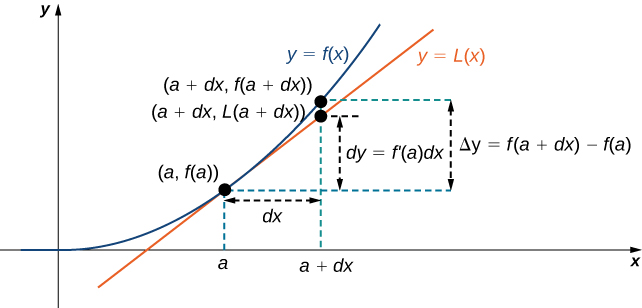Figure 5. The differential $dy={f}^{\prime } (a)dx$ is used to approximate the actual change in $y$ if $x$ increases from $a$ to $a+dx.$

We now take a look at how to use differentials to approximate the change in the value of the function that results from a small change in the value of the input. Note the calculation with differentials is much simpler than calculating actual values of functions and the result is very close to what we would obtain with the more exact calculation.

### Approximating Change with Differentials

Let $y={x}^{2}+2x.$ Compute $\Delta y$ and dy at $x=3$ if $dx=0.1.$

#### Solution

The actual change in $y$ if $x$ changes from $x=3$ to $x=3.1$ is given by

$\Delta y=f(3.1)-f(3)=\left[{(3.1)}^{2}+2(3.1)\right]-\left[{3}^{2}+2(3)\right]=0.81.$

The approximate change in $y$ is given by $dy={f}^{\prime } (3)dx.$ Since ${f}^{\prime } (x)=2x+2,$ we have

$dy={f}^{\prime } (3)dx=(2(3)+2)(0.1)=0.8.$

For $y={x}^{2}+2x,$ find $\Delta y$ and $dy$ at $x=3$ if $dx=0.2.$

#### Hint

$dy={f}^{\prime } (3)dx,$ $\Delta y=f(3.2)-f(3)$

#### Solution

$dy=1.6,$ $\Delta y=1.64$

# Calculating the Amount of Error

Any type of measurement is prone to a certain amount of error. In many applications, certain quantities are calculated based on measurements. For example, the area of a circle is calculated by measuring the radius of the circle. An error in the measurement of the radius leads to an error in the computed value of the area. Here we examine this type of error and study how differentials can be used to estimate the error.

Consider a function $f$ with an input that is a measured quantity. Suppose the exact value of the measured quantity is $a,$ but the measured value is $a+dx.$ We say the measurement error is dx (or $\Delta x).$ As a result, an error occurs in the calculated quantity $f(x).$ This type of error is known as a propagated error and is given by

$\Delta y=f(a+dx)-f(a).$

Since all measurements are prone to some degree of error, we do not know the exact value of a measured quantity, so we cannot calculate the propagated error exactly. However, given an estimate of the accuracy of a measurement, we can use differentials to approximate the propagated error $\Delta y.$ Specifically, if $f$ is a differentiable function at $a,$ the propagated error is

$\Delta y\approx dy={f}^{\prime } (a)dx.$

Unfortunately, we do not know the exact value $a.$ However, we can use the measured value $a+dx,$ and estimate

$\Delta y\approx dy\approx {f}^{\prime } (a+dx)dx.$

In the next example, we look at how differentials can be used to estimate the error in calculating the volume of a box if we assume the measurement of the side length is made with a certain amount of accuracy.

### Volume of a Cube

Suppose the side length of a cube is measured to be 5 cm with an accuracy of 0.1 cm.

1. Use differentials to estimate the error in the computed volume of the cube.
2. Compute the volume of the cube if the side length is (i) 4.9 cm and (ii) 5.1 cm to compare the estimated error with the actual potential error.

#### Solution

1. The measurement of the side length is accurate to within $\pm 0.1$ cm. Therefore,
$-0.1\le dx\le 0.1.$

The volume of a cube is given by $V={x}^{3},$ which leads to

$dV=3{x}^{2}dx.$

Using the measured side length of 5 cm, we can estimate that

$-3{(5)}^{2}(0.1)\le dV\le 3{(5)}^{2}(0.1).$

Therefore,

$-7.5\le dV\le 7.5.$
2. If the side length is actually 4.9 cm, then the volume of the cube is
$V(4.9)={(4.9)}^{3}=117.649{\text{cm}}^{3}.$

If the side length is actually 5.1 cm, then the volume of the cube is

$V(5.1)={(5.1)}^{3}=132.651{\text{cm}}^{3}.$

Therefore, the actual volume of the cube is between 117.649 and 132.651. Since the side length is measured to be 5 cm, the computed volume is $V(5)={5}^{3}=125.$ Therefore, the error in the computed volume is

$117.649-125\le \Delta V\le 132.651-125.$

That is,

$-7.351\le \Delta V\le 7.651.$

We see the estimated error $dV$ is relatively close to the actual potential error in the computed volume.

Estimate the error in the computed volume of a cube if the side length is measured to be 6 cm with an accuracy of 0.2 cm.

#### Hint

$dV=3{x}^{2}dx$

#### Solution

The volume measurement is accurate to within $21.6{\text{cm}}^{3}.$

The measurement error dx $(\text{=Δ}x)$ and the propagated error $\Delta y$ are absolute errors. We are typically interested in the size of an error relative to the size of the quantity being measured or calculated. Given an absolute error $\Delta q$ for a particular quantity, we define the relative error as $\frac{\Delta q}{q},$ where $q$ is the actual value of the quantity. The percentage error is the relative error expressed as a percentage. For example, if we measure the height of a ladder to be 63 in. when the actual height is 62 in., the absolute error is 1 in. but the relative error is $\frac{1}{62}=0.016,$ or $1.6\%.$ By comparison, if we measure the width of a piece of cardboard to be 8.25 in. when the actual width is 8 in., our absolute error is $\frac{1}{4}$ in., whereas the relative error is $\frac{0.25}{8}=\frac{1}{32},$ or $3.1\%.$ Therefore, the percentage error in the measurement of the cardboard is larger, even though 0.25 in. is less than 1 in.

### Relative and Percentage Error

An astronaut using a camera measures the radius of Earth as 4000 mi with an error of $\pm 80$ mi. Let’s use differentials to estimate the relative and percentage error of using this radius measurement to calculate the volume of Earth, assuming the planet is a perfect sphere.

#### Solution

If the measurement of the radius is accurate to within $\pm 80,$ we have

$-80\le dr\le 80.$

Since the volume of a sphere is given by $V=(\frac{4}{3})\pi {r}^{3},$ we have

$dV=4\pi {r}^{2}dr.$

Using the measured radius of 4000 mi, we can estimate

$-4\pi {(4000)}^{2}(80)\le dV\le 4\pi {(4000)}^{2}(80).$

To estimate the relative error, consider $\frac{dV}{V}.$ Since we do not know the exact value of the volume $V,$ use the measured radius $r=4000\text{mi}$ to estimate $V.$ We obtain $V\approx (\frac{4}{3})\pi {(4000)}^{3}.$ Therefore the relative error satisfies

$\frac{-4\pi {(4000)}^{2}(80)}{4\pi {(4000)}^{3}\text{/}3}\le \frac{dV}{V}\le \frac{4\pi {(4000)}^{2}(80)}{4\pi {(4000)}^{3}\text{/}3},$

which simplifies to

$-0.06\le \frac{dV}{V}\le 0.06.$

The relative error is 0.06 and the percentage error is $6\%.$

Determine the percentage error if the radius of Earth is measured to be 3950 mi with an error of $\pm 100$ mi.

#### Hint

Use the fact that $dV=4\pi {r}^{2}dr$ to find $dV\text{/}V.$

7.6%

### Key Concepts

• A differentiable function $y=f(x)$ can be approximated at $a$ by the linear function
$L(x)=f(a)+{f}^{\prime } (a)(x-a).$
• For a function $y=f(x),$ if $x$ changes from $a$ to $a+dx,$ then
$dy={f}^{\prime } (x)dx$

is an approximation for the change in $y.$ The actual change in $y$ is

$\Delta y=f(a+dx)-f(a).$
• A measurement error $dx$ can lead to an error in a calculated quantity $f(x).$ The error in the calculated quantity is known as the propagated error . The propagated error can be estimated by
$dy\approx {f}^{\prime } (x)dx.$
• To estimate the relative error of a particular quantity $q,$ we estimate $\frac{\Delta q}{q}.$

# Key Equations

• Linear approximation
$L(x)=f(a)+{f}^{\prime } (a)(x-a)$
• A differential
$dy={f}^{\prime } (x)dx.$

1. What is the linear approximation for any generic linear function $y=mx+b?$

2. Determine the necessary conditions such that the linear approximation function is constant. Use a graph to prove your result.

#### Solution

${f}^{\prime }(a)=0$

3. Explain why the linear approximation becomes less accurate as you increase the distance between $x$ and $a.$ Use a graph to prove your argument.

4. When is the linear approximation exact?

#### Solution

The linear approximation exact when $y=f(x)$ is linear or constant.

For the following exercises, find the linear approximation $L(x)$ to $y=f(x)$ near $x=a$ for the function.

5. [T] $f(x)=x+{x}^{4},a=0$

6. [T] $f(x)=\frac{1}{x},a=2$

#### Solution

$L(x)=\frac{1}{2}-\frac{1}{4}(x-2)$

7. [T] $f(x)= \tan x,a=\frac{\pi }{4}$

8. [T] $f(x)= \sin x,a=\frac{\pi }{2}$

#### Solution

$L(x)=1$

9. [T] $f(x)=x \sin x,a=2\pi$

10. [T] $f(x)={ \sin }^{2}x,a=0$

#### Solution

$L(x)=0$

For the following exercises, compute the values given within 0.01 by deciding on the appropriate $f(x)$ and $a,$ and evaluating $L(x)=f(a)+{f}^{\prime }(a)(x-a).$ Check your answer using a calculator.

11. [T] ${(2.001)}^{6}$

12. [T] $\sin (0.02)$

#### Solution

0.02

13. [T] $\cos (0.03)$

14. [T] ${(15.99)}^{1\text{/}4}$

#### Solution

1.9996875

15. [T] $\frac{1}{0.98}$

16. [T] $\sin (3.14)$

#### Solution

0.001593

For the following exercises, determine the appropriate $f(x)$ and $a,$ and evaluate $L(x)=f(a)+{f}^{\prime }(a)(x-a).$ Calculate the numerical error in the linear approximations that follow.

17. ${(1.01)}^{3}$

18. $\cos (0.01)$

#### Solution

$1;$ error, $~0.00005$

19. ${( \sin (0.01))}^{2}$

20. ${(1.01)}^{-3}$

#### Solution

$0.97;$ error, $~0.0006$

21. ${(1+\frac{1}{10})}^{10}$

22. $\sqrt{8.99}$

#### Solution

$3-\frac{1}{600};$ error, $~4.632×{10}^{-7}$

For the following exercises, find the differential of the function.

23. $y=3{x}^{4}+{x}^{2}-2x+1$

24. $y=x \cos x$

#### Solution

$dy=( \cos x-x \sin x)dx$

25. $y=\sqrt{1+x}$

26. $y=\frac{{x}^{2}+2}{x-1}$

#### Solution

$dy=(\frac{{x}^{2}-2x-2}{{(x-1)}^{2}})dx$

For the following exercises, find the differential and evaluate for the given $x$ and $dx.$

27. $y=3{x}^{2}-x+6,$ $x=2,$ $dx=0.1$

28. $y=\frac{1}{x+1},$ $x=1,$ $dx=0.25$

#### Solution

$dy=-\frac{1}{{(x+1)}^{2}}dx,$ $-\frac{1}{16}$

29. $y= \tan x,$ $x=0,$ $dx=\frac{\pi }{10}$

30. $y=\frac{3{x}^{2}+2}{\sqrt{x+1}},$ $x=0,$ $dx=0.1$

#### Solution

$dy=\frac{9{x}^{2}+12x-2}{2{(x+1)}^{3\text{/}2}}dx,$ $-0.1$

31. $y=\frac{ \sin (2x)}{x},$ $x=\pi ,$ $dx=0.25$

32. $y={x}^{3}+2x+\frac{1}{x},$ $x=1,$ $dx=0.05$

#### Solution

$dy=(3{x}^{2}+2-\frac{1}{{x}^{2}})dx,$ $0.2$

For the following exercises, find the change in volume $dV$ or in surface area $dA.$

33. $dV$ if the sides of a cube change from 10 to 10.1.

34. $dA$ if the sides of a cube change from $x$ to $x+dx.$

#### Solution

$12xdx$

35. $dA$ if the radius of a sphere changes from $r$ by $dr.$

36. $dV$ if the radius of a sphere changes from $r$ by $dr.$

#### Solution

$4\pi {r}^{2}dr$

37. $dV$ if a circular cylinder with $r=2$ changes height from 3 cm to $3.05\text{cm}.$

38. $dV$ if a circular cylinder of height 3 changes from $r=2$ to $r=1.9\text{cm}.$

#### Solution

$-1.2\pi {\text{cm}}^{3}$

For the following exercises, use differentials to estimate the maximum and relative error when computing the surface area or volume.

39. A spherical golf ball is measured to have a radius of $5\text{mm},$ with a possible measurement error of $0.1\text{mm}.$ What is the possible change in volume?

40. A pool has a rectangular base of 10 ft by 20 ft and a depth of 6 ft. What is the change in volume if you only fill it up to 5.5 ft?

#### Solution

-100 ft 3

41. An ice cream cone has height 4 in. and radius 1 in. If the cone is 0.1 in. thick, what is the difference between the volume of the cone, including the shell, and the volume of the ice cream you can fit inside the shell?

For the following exercises, confirm the approximations by using the linear approximation at $x=0.$

42. $\sqrt{1-x}\approx 1-\frac{1}{2}x$

43. $\frac{1}{\sqrt{1-{x}^{2}}}\approx 1$

44. $\sqrt{{c}^{2}+{x}^{2}}\approx c$

## Glossary

differential
the differential $dx$ is an independent variable that can be assigned any nonzero real number; the differential $dy$ is defined to be $dy={f}^{\prime } (x)dx$
differential form
given a differentiable function $y={f}^{\prime } (x),$ the equation $dy={f}^{\prime } (x)dx$ is the differential form of the derivative of $y$ with respect to $x$
linear approximation
the linear function $L(x)=f(a)+{f}^{\prime } (a)(x-a)$ is the linear approximation of $f$ at $x=a$
percentage error
the relative error expressed as a percentage
propagated error
the error that results in a calculated quantity $f(x)$ resulting from a measurement error dx
relative error
given an absolute error $\Delta q$ for a particular quantity, $\frac{\Delta q}{q}$ is the relative error.
tangent line approximation (linearization)
since the linear approximation of $f$ at $x=a$ is defined using the equation of the tangent line, the linear approximation of $f$ at $x=a$ is also known as the tangent line approximation to $f$ at $x=a$